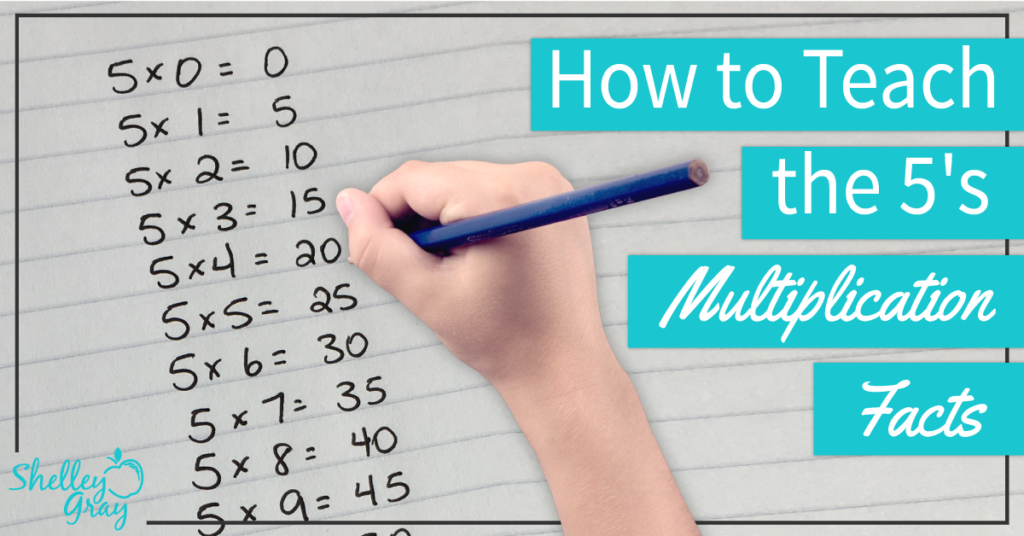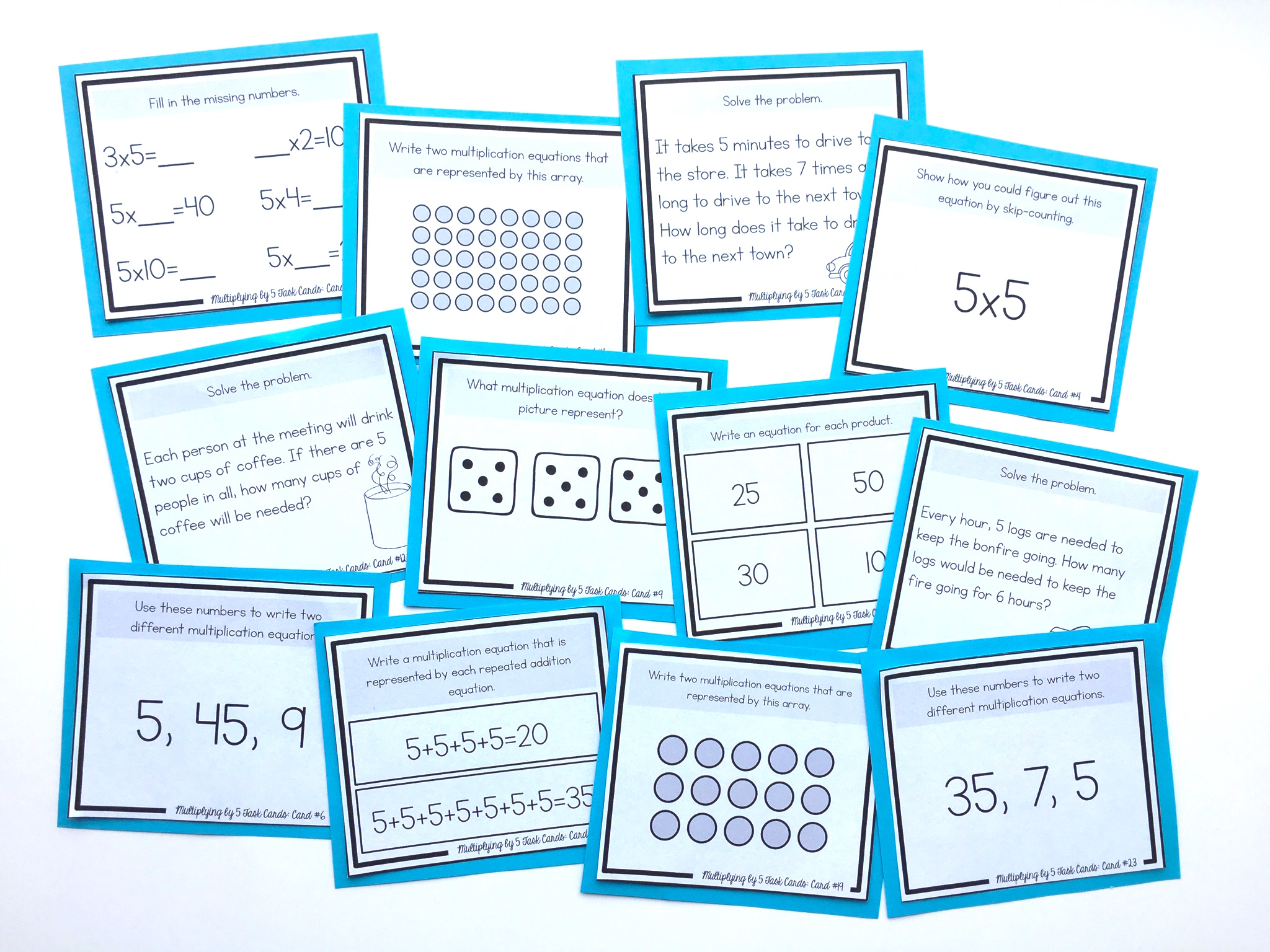# How to Teach the 5 Times Table So It Really Makes SenseI usually find that students memorize the 5’s multiplication facts fairly easily. There are a couple of ways to help them learn these facts.

First of all, you can teach students to first multiply by 10, and then divide that product in half.

For example, when you have an equation like 5×8, you could first do 10×8 to make 80, and then divide that in half to make 40. The reason that this works is because 5 is half of 10.

As another example, for the equation 5×4, you could first multiply 10×4 to make 40, and then divide that product in half to make 20.

The strategy above is a good one to encourage number-sense understanding of the 5 times table. Arrays are an excellent visual to show the connection between 5 and 10.

In my own experience I have found that skip-counting is a quick and effective strategy for this times table, since students generally memorize the products quickly anyways.

For example, for 5×3, skip-count by 5’s three times: 5, 10, 15.

For 5×6, skip-count by 5’s six times: 5, 10, 15, 20, 25, 30.

For both of these strategies, it is very important to reinforce the commutative property.

This means that the order of factors does not change the product. For example, if students are faced with an equation like 4×5, they should see the 5 as one of the factors, and know that they can use the 5’s strategy to solve this equation.

NEXT STEPS

Reinforce the 5’s multiplication facts with this set of task cards. Students will learn conceptually through problem-solving, using arrays, strategic thinking, finding missing numbers, skip-counting, picture representations, and more:OR FIND THE FULL BUNDLE OF MULTIPLICATION TASK CARDS HERE.